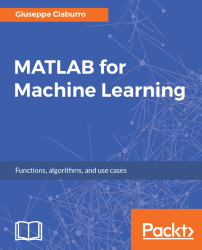•#### MATLAB for Machine Learning#### Overview of this book

MATLAB is the language of choice for many researchers and mathematics experts for machine learning. This book will help you build a foundation in machine learning using MATLAB for beginners. You’ll start by getting your system ready with t he MATLAB environment for machine learning and you’ll see how to easily interact with the Matlab workspace. We’ll then move on to data cleansing, mining and analyzing various data types in machine learning and you’ll see how to display data values on a plot. Next, you’ll get to know about the different types of regression techniques and how to apply them to your data using the MATLAB functions. You’ll understand the basic concepts of neural networks and perform data fitting, pattern recognition, and clustering analysis. Finally, you’ll explore feature selection and extraction techniques for dimensionality reduction for performance improvement. At the end of the book, you will learn to put it all together into real-world cases covering major machine learning algorithms and be comfortable in performing machine learning with MATLAB.
Title PageCreditsForewordwww.PacktPub.comCustomer FeedbackPrefaceFree Chapter
Getting Started with MATLAB Machine LearningImporting and Organizing Data in MATLABFrom Data to Knowledge DiscoveryFinding Relationships between Variables - Regression TechniquesPattern Recognition through Classification AlgorithmsIdentifying Groups of Data Using Clustering MethodsSimulation of Human Thinking - Artificial Neural NetworksImproving the Performance of the Machine Learning Model - Dimensionality ReductionMachine Learning in Practice## Summary

In this chapter, we explored the amazing world of machine learning and took a tour of the most popular machine learning algorithms to choose the right one for our needs. To understand what is most suitable for our needs, we learned to perform a preliminary analysis. Then we analyzed how to build machine learning models step by step.

Afterwards, we discovered the machine learning capabilities in MATLAB for classification, regression, clustering, and deep learning, including apps for automated model training and code generation. We verified the MATLAB system requirements and platform availability for a correct installation.

Finally, we introduced the Statistics and Machine Learning Toolbox and Neural Network Toolbox. We learned what we can do with these tools, and what algorithms we need to use to solve our problems. We understood the role of statistics and algebra in machine learning and how MATLAB can help us.

In the next chapter, we will learn how to easily interact with the MATLAB workspace, import and organize our data in MATLAB, export data from the workspace, and organize the data in the correct format for the next phase of data analysis.